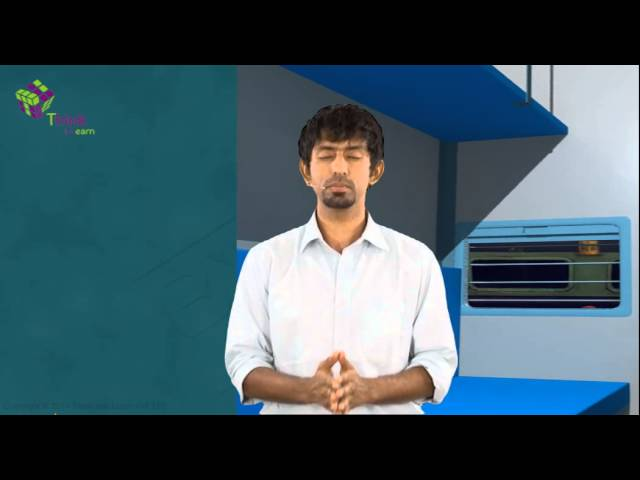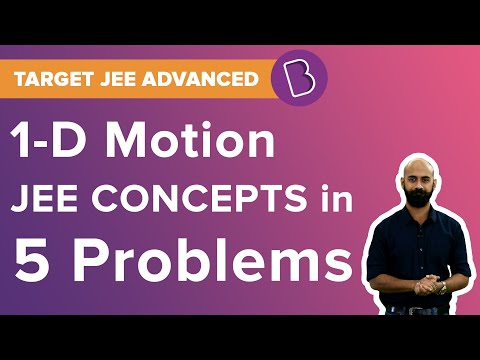# Class 11 Physics Chapter 3 Notes Motion In A Straight Line

## Motion in a Straight Line

A body is said to be in motion if it changes its position with respect to time.

To get more details on Motion in a Straight Line, visit here.### Types of Rectilinear/Linear Motion

The Rectilinear Motion or linear motion can be of two types:

1. Non-Uniform linear motion with non-zero acceleration or variable velocity
2. Uniform linear motion with zero acceleration or constant velocity

The simplest type of one-dimensional motion is Linear motion. As per Newton’s first law of motion, an object will either be in rest or continue to move in a straight line with a uniform velocity unless and until an external force is applied to it.

Linear motion is often mistaken for general motion. Linear motion is a one-dimensional motion but in general, the motion has both magnitude and direction, i.e. an object’s position and velocity are described in vector quantities.

To get more details on Rectilinear Motion, visit here.

### Uniform Motion in a Straight LineA body is said to be in a uniform motion if it travels in a straight line covering equal distances in equal intervals of time. A body is said to have uniform acceleration if the rate of change of its velocity remains constant.

The ball covers equal distances in equal intervals of time

Example:

If a car travels at a speed of 60 km/hour then it will cover a distance of 1 km/minute. In this sense, the motion of car acceleration is uniform.

#### For More Information On Motion in One Dimension, Watch The Below Video:To get more details on Motion in a Straight Line, visit here.

### Non-uniform Motion in a Straight Line

A body is said to have a non-uniform motion when the velocity of a body changes by unequal amounts in equal intervals of time. While in movement, the rate of change of its velocity changes at different points of time.

Example:

A boy kicking a football. It might cover 4 meters in the first attempt, 6 meters in the second change, 9 meters in the third attempt and so on as per the velocity exerted by the boy.

To get more details on Uniform and Non-Uniform Motion, visit here.

### Path Length

The path length is the total length of the path that has to be traversed by the object.

### Displacement

Displacement is the change in position of a body. The magnitude of displacement between the same points is proportional to the path length.

To get more details on Displacement Formula, visit here.

### Uniform Motion

When the displacement of an object is equal in equal time intervals, the motion is said to be uniform, else it is referred to as non-uniform.

### Average Speed

Average speed is referred to the ratio between the total path length traversed to the time taken.

To get more details on Average Speed, visit here.

### Instantaneous Velocity

It is the velocity when the limit of the average velocity has an indefinitely smaller interval of time.

To get more details on Instantaneous Velocity Formula, visit here.

### Average Acceleration

It is the change in velocity corresponding to the time interval with in which the change has accelerated.

### Important Questions

1. In which of the following examples of motion, can the body be considered approximately a point object:
• (a) a tumbling beaker that has slipped off the edge of a table
• (b) a monkey sitting on top of a man cycling smoothly on a circular track.
• (c) a railway carriage moving without jerks between two stations.(d)a spinning cricket ball that turns sharply on hitting the ground
1. The position-time (x-t) graphs for two children A and B returning from their school O to their homes P and Q respectively are shown in Fig. 3.19. Choose the correct entries in the brackets below ;
• (a) (A/B) lives closer to the school than (B/A)
• (b) (A/B) overtakes (B/A) on the road (once/twice)
• (c) (A/B) walks faster than (B/A)
• (d) A and B reach home at the (same/different) time
• (e) (A/B) starts from the school earlier than (B/A)

Visit the links below to get the class 11 physics chapter 3 (Motion in a Straight Line) notes. Motion in a Straight Line NCERT Solution Motion in a Straight Line

Visit BYJU’S for chapter wise CBSE notes of all the classes. At BYJU’S we also provide latest CBSE Sample Papers, Question Papers, and Study Materials.

## Frequently asked Questions on CBSE Class 11 Physics Notes Chapter 3: Motion in a Straight Line

### What is difference between 1D and 2D motion?

1D motion means motion in One dimension. That is the body moves only in one axis either x y or Z. Or simply it moves only linearly. Whereas 2D motion involves motion which is defined as the combination between two axes.

### What is non-uniform motion?

Non-uniform motion is the motion of an object wherein the object moves at different speeds and does not cover the same distance at equal time intervals.

### What is ‘Acceleration’?

The rate at which velocity changes with time, in terms of both speed and direction is called as acceleration.